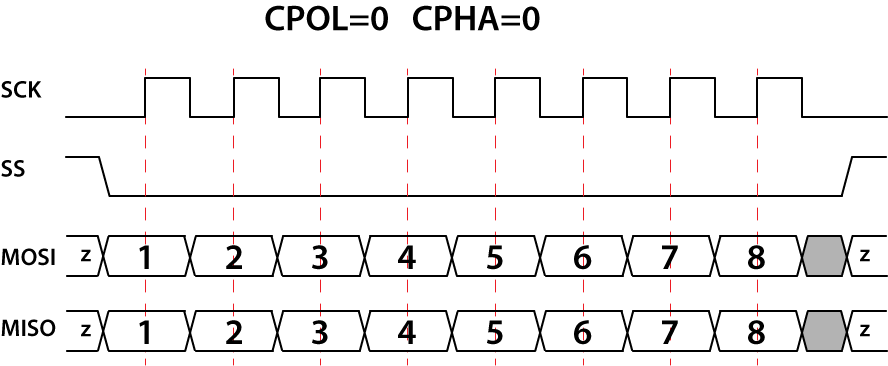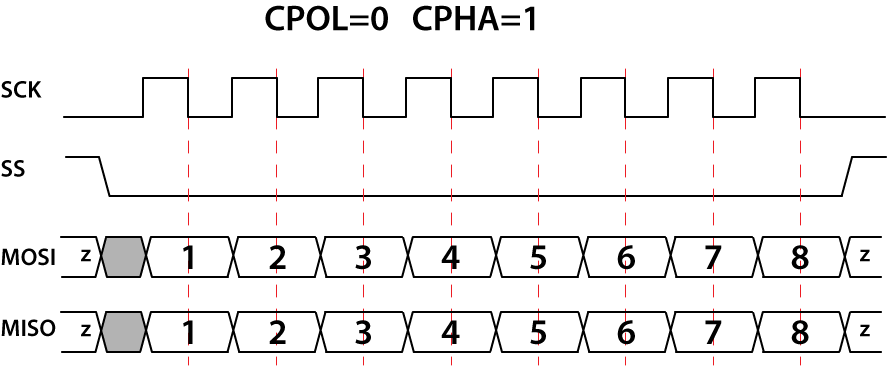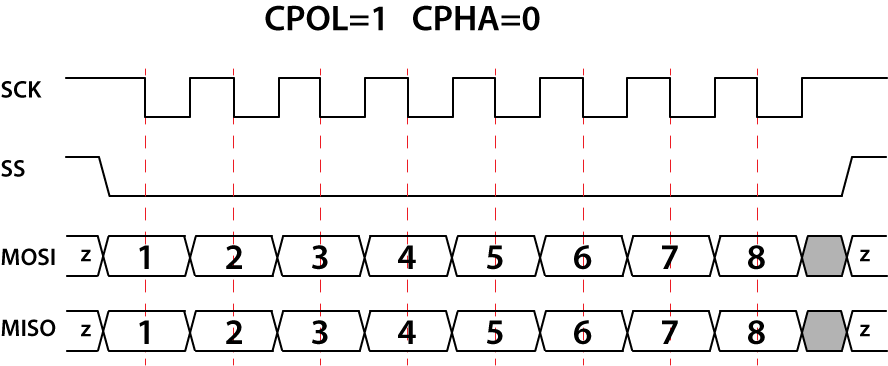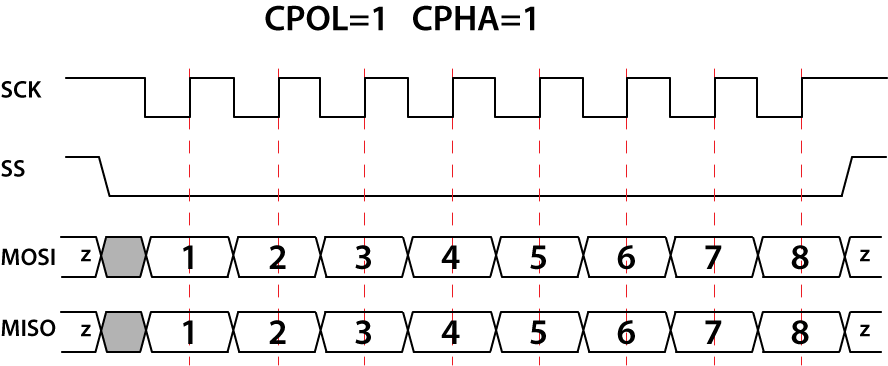# Clock Phase and Polarity

The master configures the clock polarity (CPOL) and clock phase (CPHA) to correspond to slave device requirements. These parameters determine when the data must be stable, when it should be changed according to the clock line and what the clock level is when the clock is not active.

The CPOL parameter assigns the clock level when the clock is not active. The clock (SCK) signal may be inverted (CPOL=1) or non-inverted (CPOL=0). For the inverted clock signal, the first clock edge is falling. For the non-inverted clock signal, the first clock edge is rising.

The CPHA parameter is used to shift the capturing phase. If CPHA=0, the data are captured on the leading (first) clock edge, regardless of whether that clock edge is rising or falling. If CPHA=1, the data are captured on the trailing (second) clock edge; in this case, the data must be stable for a half cycle before the first clock cycle.

There are four possible modes that can be used in an SPI protocol:

1. For CPOL=0, the base value of the clock is zero. For CPHA=0, data are captured on the clock’s rising edge and data are propagated on a falling edge.2. For CPOL=0, the base value of the clock is zero. For CPHA=1, data are captured on the clock’s falling edge and data are propagated on a rising edge.3. For CPOL=1, the base value of the clock is one. For CPHA=0, data are captured on the clock’s rising edge and data are propagated on a falling edge.4. For CPOL=1, the base value of the clock is one. For CPHA=1, data are captured on the clock’s falling edge and data are propagated on a rising edge.In DLN adapters, the default transmission mode configuration has CPOL=0, CPHA=0 values.

You can specify the mode using the DlnSpiMasterSetMode() function. To configure the CPOL and CPHA values separately, use the DlnSpiMasterSetCpol() and DlnSpiMasterSetCpha() functions.

The master and a slave must use the same set of parameters (clock frequency, CPOL and CPHA); otherwise, a communication will be impossible. If multiple slaves are used, the master configuration should change each time before the master initiates communication with a different slave.

Rating: